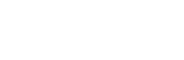#fuli2020

2020-08-20   阅读量: 158

## 机器学习——决策树

1. 根节点：包含样本全集。没有入边，但有零条或多条出边；

2. 内部结点：对应于属性测试条件，恰有一条入边，和两条或多条出边

3. 叶结点：对应于决策结果，恰有一条入边，但没有出边。

1. 香农熵2.信息增益3.划分数据集

1. 利用ID3算法建立决策树

ID3算法的核心是在决策树各个结点应用信息增益准则选择特征，递归地构建决策树。具体方法是：

1）从根结点开始，对结点计算所有可能的特征的信息增益

2）选择信息增益最大的特征作为结点的特征，由该特征的不同取值建立子结点

3）再对子结点调用以上方法，构建决策树

4）直到所有特征的信息增益均很小或没有特征可以选择为止，最后得到一个决策树

ID3算法局限主要源于局部最优化条件，即信息增益的计算⽅法，其局限性主要有以下⼏点：
1）分⽀度越⾼（分类⽔平越多）的离散变量往往⼦结点的总信息熵更⼩，ID3是按照某⼀列进⾏切

2）不能直接处理连续型变量，若要使⽤ID3处理连续型变量，则⾸先需要对连续变量进⾏离散化。

3）没有剪枝的设置，容易导致过拟合，即在训练集上表现很好，测试集上表现很差

C4.5算法

C4.5在生成的过程中，用信息增益比准则选择特征CART算法：分类回归树

1）分裂过程是一个二叉递归划分过程

2）CART预测变量x的类型既可以是连续型变量也可以是分类型变量

3）数据应以其原始形式处理，不需要离散化

4）用于数值型预测时，并没有使用回归，而是基于到达叶结点的案例的平均值做出预测

1. 分裂准则class sklearn.tree.DecisionTreeClassifier(criterion='gini',splitter='best',max_depth=None,min_samples_split=2,min_samples_leaf=1,min_weight_fraction_leaf=0,max_features=None,random_state=None,max_leaf_nodes=None,min_impurity_decrease=0,min_impurity_split=None,class_weight=None,presort=False)

1) criterion

criterion这个参数是用来决定不纯度的计算方法，sklearn提供了两种选择：

2）random_state & splitter

random_state用来设置分支中的随机模式的参数，默认None,在高纬度时随机性会表现更明显。、

splitter也是用来控制决策树中的随机选项的，有两种输入值，输入“best”,决策树会有限选择更重要的特征进行分支（重要性可以通过属性feature_importances_查看），输入“random”决策树在分支时会更加随机，树会因为含有更多的不必要信息而更深更大，并因这些不必要信息而降低对训练集的拟合，这也是防止过拟合的一种方式。

3）剪枝参数

max_depth：限制树的最大深度，超过设定深度的树枝全部剪掉，建议从=3开始尝试，看看拟合的效果再决定是否增加设定深度

min_samples_leaf: 一个结点在分支后的每个子节点都必须包含至少min_samples_leaf个训练样本，否则分支就不会发生。一般来说，建议从=5开始使用，如果叶结点中含有的样本量变化很大，建议输入浮点数作为样本量的百分比来使用。对于类别不多的分类问题，=1通常就是最佳选择

min_samples_split限定，一个结点必须要包含至少min_samples_split个训练样本，这个结点才允许被分支，否则分支就不会发生

sklearn决策树最常用的接口还有apply和predict。apply中输入测试集返回每个测试样本所在的叶子结点的索引，predict输入测试集返回每个测试样本的标签。所有接口中要求输入Xtrain和Xtest的部分，输入的特征矩阵必须至少是一个二维矩阵。

1. 二分类决策树中的样本不均衡问题。

样本不均衡是指在一组数据集中，标签的一类天生占有很大的比例，但我们有着捕捉出某种特定的分类的需求的状况。

在决策树中，存在着调节样本均衡的参数：class_weight和接口fit中可以设定得sample_weight。

在决策树中，参数class_weight默认None，此模式表示假设数据集中的所有标签是均衡的，即⾃动认为
标签的⽐例是1：1。所以当样本不均衡的时候，我们可以使⽤形如{"标签的值1"：权重1，"标签的值
2"：权重2}的字典来输⼊真实的样本标签⽐例，来让算法意识到样本是不平衡的。或者使
⽤”balanced“模式，直接使⽤n_samples/(n_classes * np.bincount(y))作为权重，可以⽐较好地修正我
们的样本不均衡情况。TP：真实为1，预测也为1

FN：真实为1，预测为0

FP：真实为0，预测为1

TN：真实为0，预测也为0

Accuracy=(TP+TN)/(TP+TN+FP+FN)

Precision=TP/(TP+FP)

Sensitivity=TP/(TP+FN)

F-measure=2/(1/precision+1/Recall)=2precision*recall/(precision+recall)

sklearn 中的混淆矩阵

sklearn.metrics.confusion_matrix 混淆矩阵

sklearn.metrics.accuracy_score 准确度accuracy

sklearn.metrics.precision_score 精确度precision

sklearn.metrics.recall_score 召回率recall

sklearn.metrics.precision_recall_curve 精确度-召回率平衡曲线

sklearn.metrics.f1_score F1 measure

#做学习曲线，观测最好的maxdepth

#对训练集使用交叉验证

from sklearn.model_selection import cross_val_score

l=[]

for i in range(1,101):

clf=DecisionTreeClassifier(max_depth=i,

splitter='best'

)

l.append(cross_val_score(clf,Xtrain,Ytrain,cv=10).mean())

plt.plot(range(1,101),l,label='max_depth')

plt.legend()

plt.show()

#网格搜索

from sklearn.model_selection import GridSearchCV

import numpy as np

#首先确定一个网格

#字典的形式，Key为决策树的参数，值为一个范围，需要是数组的形式

parameters={

'max_depth':[1,3,4,5,6],

'min_samples_leaf':[3,4,5,6,7,8,9,10],

'splitter':['best']

class_weight=[{1:10,0:1},

{1:9,0:1},

{1:8,0:1}

]

}

#实例化一个默认的决策树

#不用自己设定任何参数的，网格搜索包会自动将所有的字典当中可能的组自动传进去

clf=DecisionTreeClassifier()

#造一个GS对象，只需要定义好的模型，和参数字典，cv

GS=GridSearchCV(clf,parameters,cv=5)

#属性best_params_查看调整出来的最佳参数

print(GS.best_params_)

#属性best_score_查看在最好的参数下，交叉验证中小测试集上最高的一个表现

print(GS.best_score_)

#最好的参数已经存到这个GS，这个已经是最好的一个决策树，直接可以来使用score

GS.score(Xtrain,Ytrain),GS.score(Xtest,Ytest)

#如果提前不知道是否有数据不平衡，首先观测标签占比

#如果发现数据不平衡，一上来在实例化的时候就要开始加

pd.Series(y).value_counts()

#加权重

wclf=DecisionTreeClassifier(max_depth=4,

#class_weight={1:10,0:1},

class_weight='balanced'

)

wclf.fit(X,y)

print(wclf.score(X,y))

#专门的一个sklearn包，做模型评估的

from sklearn.metrics import recall_score,precision_score,f1_score

y_pred=clf.predict(X)

print(recall_score(y,y_pred),precision_score(y,y_pred),f1_score(y,y_pred))

#1.读入数据

import numpy as np

import pandas as pd

#了解数据

df.info()

#删掉ID这一列

df.drop('KBM_INDV_ID',axis=1,inplace=True)

label=df.pop('resp_flag')

df['label']=label

#记录最初的数据类型，并保存下来，方便后面进行对比

df_org=df.copy()

df.describe().T

#统计一下每一列中有多少个空值

#检查是否有缺失的列

NA=df.isnull().mean()

NA=NA.reset_index()

NA.columns=['features','Missing_count']

#过滤出大于0的数据

NA=NA[NA['Missing_count']>0].reset_index(drop=True)

#3 查看数据中是否有重复值

df[df.duplicated()]

#df.drop_duplicates(inplace=True)

#4. 可视化数据查看数据分布情况

#引入画图模块

import seaborn as sns

import matplotlib.pyplot as plt

import numpy as np

plt.style.use('seaborn')

#支持中文

plt.rcParams['font.sans-serif']=['SimHei']

plt.rcParams['axes.unicode_minus']=False

#4.1 看一下目标变量是否平衡

#查看性别比例

plt.figure(1,figsize=(10,3))

sns.countplot(y='label',data=df)

plt=show()

#统计购买用户和未购买用户的比例关系

df['label'].value_counts()/df.shape

sns.distplot(dfs['age'],bins=20)

#4.3 分别绘制两类样本的年龄分布

# 为连续型变量：年龄创建密度图

plt.xlim(([60,90]))

plt.xlabel('age')

plt.ylabel('Density')

#查看性别比例

plt.figure(1,figsize=(10,3))

sns.countplot(y='GEND',data=df)

plt.show()

sns.countplot(x='GEND',hue='label',data=df)

plt.xlabel('GEND')

plt.ylabel('Amount')

#学历情况

df.c210mys.value_counts()

#查看学历分布情况

plt.figure(1,figsize=(10,3))

sns.countplot(y='c210mys',data=df)

plt.show()

sns.countplot(x='c210mys',hue='label',data=df)

plt.xlabel('学历')

plt.ylabel('购买数量')

#县级别和购买比例

sns.countplot(x='N2NCY',hue='label',data=df)

plt.xlabel('县的大小')

plt.ylabel('购买数量')

#5 空值填充

NA['features']

df.dtypes[NA['features']].values

# 通过索引来提取有空缺值得这些特征的类型

NA['数据类型']=df.dtypes[NA['features']].values

#决定除年龄之外的其他特征，我们采取众数进行填充，年龄采取均值进行填充

def fillna_apply(i):

global df

colname=i.values

if colname!='age':

df[colname].fillna(df[colname].mode(),inplace=True)

else:

df[colname].fillna(df[colname].mean(),inplace=True)

print('column {} is filled'.format(colname))

#对这些列名进行遍历，依次进行填充

NA.apply(lambda i:fillna_apply(i),axis=1)

#5.3 确认是否全部填充完成

df.isnull().sum().sum()

#6，变量编码

#首先把用户ID列删除

#del df['KBM_INDV_ID']

object_cols=df.dtypes[df.dtypes=='object'].index.tolist()

#对任意一个object类型的特征，来做编码的函数

def encode(col_name):

col=df[col_name]

#算出unique的值

levels=col.unique()

#计算出这个的长度，使用range来生成一个list

saize=range(len(levels))

#使用zip加上列表解析的方式来进行字典的创建

d={i : j for i,j in zip(levels,size)}

df[col_name].replace(d,inplace=True)

#对每一个是object的特征来进行编码

for col_name in object_cols:

encode(col_name)

#7.1 切分数据集

#将数据集切分成训练数据和测试数据

from sklearn import tree

from sklearn.model_selection import train_test_split

from sklearn.metrics import classification_report,roc_auc_score

from sklearn import metrics

from sklearn.model_selection import cross_val_score

from sklearn.model_selection import GridSearchCV

import matplotlib.pylab as plt

from matplotlib.pylab import rcParams

#取出x和y

x=df.iloc[:,:-1]

y=df.iloc[:,-1]

#将数据集7:3分，70%用来建模，30%用来测试

X_train,X_test,y_train,y_test=train_test_split(x,y,test_size=0.3,random_state=66)

#用默认模型试一下

from sklearn.tree import DecisionTreeClassifier

clf=DecisionTreeClassifier()

clf.fit(X_train,y_train)

clf.score(X_train,y_train),clf.score(X_test,y_test)

clf.min_samples_split

#7.3尝试调参

clf=DecisionTreeClassifier(

class_weight=None,#指定样本各类别的权重，如果样本类别分布没有明显的偏倚，则可以不管这个参数，选择默认的“None”

criterion='gini',#特征选择标准，可以使用“gini”或者“entropy”,前者代表基尼系数，后者代表信息增益，一般说使用默认的基尼系数“gini”就可以了，即CART算法。除非你更喜欢类似ID3,C4.5的最优特征选择方法

max_depth=300,#决策树最大深，常用的可以取值10-100之间，主要是限制树的增长

max_features=None,#划分时考虑的最大特征数

max_leaf_nodes=None,#最大叶子节点数。通过限制最大叶，这个值限制了决策树的增长

min_samples_leaf=10,#叶子节点最少样本数，用于减枝

min_samples_split=10,#内部节点再划分所需最小样本数，这个值限制了子树继续划分的条件

min_weight_fraction_leaf=0.0,#叶子节点最小的样本权重和，这个值限制了叶子节点所有样本权重和的最小值，如果小于这个值，则会和兄弟节点一起被剪枝。默认是0，就是不考虑权重问题。一般来说，如果我们有较多样本有缺失值，或者分类树样本的分布类别偏差很大，就会引入样本权重，这时我们就要注意这个值了。

presort=False,#数据是否预排序

splitter='best'#特征划分点选择标准，可以使用“best”或者“random”。前者在特征的所有划分点钟找出最优的划分点。后者是随机的在部分划分点中找局部最优的划分点

)

clf.fit(X_train,y_train)

clf.score(X_train,y_train),clf.score(X_test,y_test)

#尝试使用最小叶节点样本数量和最小分割样本数量进行调参

#尝试使用最大深度和最小叶节点数量进行调参

from sklearn.ensemble import RandomForestClassifier

clf=DecisionTreeClassifier()

#clf=RandomForestClassifier()

parameters={'splitter':['best'],

'max_depth':list(range(10,30)),

'min_samples_leaf':list(range(100,300,10)),

'min_impurity_decrease':np.linspace(0,0.5,20)

}

grid=GridSearchCV(clf,parameters,cv=5)

grid.fit(X_train,y_train)

#在训练集上，模型最好的参数

grid.best_params_

#在训练集上，模型最好的分数

grid.best_score

#模型评估与混淆矩阵

y_pred=grid.predict(X_test)

from sklearn.metrics import accuracy_score,recall_score,precision_score

print('accuracy:',accuracy_score(y_test,y_pred))

print('precision:',precision_score(y_test,y_pred))

print('recall:',recall_score(y_test,y_pred))

cm=confusion_matrix(y_test,y_pred,labels=[1,0])

print('confusion matrix',cm)

36.2012 3 1 关注作者 收藏